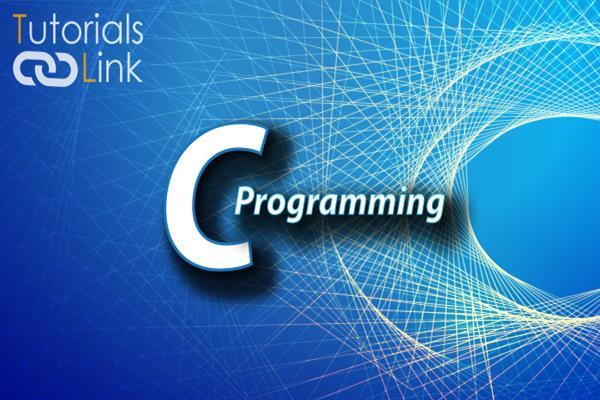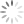Loading, please wait...# Increment And Decrement Operators in C Programming

Sep 23, 2019 Operator, c language, C Programming, 3730 Views

The C compilers produce very fast and efficient object codes for increment and decrement operations. This code is better than generated by using the equivalent assignment statement. So, increment and decrement operators should be used whenever possible. The operator ++ adds one to its operand, whereas the operator – subtracts one from its operand. For justification, x=x+1 can be written as x++-1; can be written as x--; both these operators may either follow or precede the operand. That is x=x+1;can be represented as x++; or ++x;

If ‘++’ or ‘--’ are used as a suffix to the variable name, then post-increment/decrement operations take place. Consider an example for understanding the ‘++’ operator as a prefix to the variable.

``````X=20;
y=10;
z=x*y++;``````

In the above equation, the current value of y is used for the product. The result is 200, which is assigned to ‘z’.after multiplication the value of y is increment by one.

If ‘++’ or ‘--’ are used as a prefix to the variable name then pre-increment /decrement operations take place. Consider an example for understanding ‘++’ operator as a prefix to the variable.

``````X=20;
y=10;
z=x*++y;``````

In the above equation, the value of y is incremented and then multiplication is carried out. The result is 220, which is assigned to ‘z’. The following programs can be executed for verification of increment and decrement operations.

* WAP to show the effect of increment operator as a suffix.

``````Void main ()
{
int a, z, x=10, y=20;
clrscr();
z=x*y++;
a=x*y;
printf (“\n %d”, z, a);
}``````

Output:-

``200 210``